# Second law article

From mechanics to thermodynamics
W.D. Bauer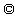released 6.7.98 little additions 11.7.98

Abstract:

It is shown that the second law is obsolete in principle because it can be derived as a consequence of the principle of least action if thetransition from mechanics to thermodynamics is made. The second law corresponds to the second variation of the least action functional and can be positive or negative according to the physical problem. Therefrom it follows that the possibility exists that the second law can reverse.

1. Introduction

Because moving particles are the deeper cause of heat, equilibrium thermodynamics should be derivable from classical mechanics principally.In this article we will present a derivation of equilibrium thermodynamics from classical mechanics. It will be shown that the extremal principle of the potentials follow from the principle of least action of classical mechanics, and therefrom the second law becomes derivable as well for concrete examples.

2. The Hamiltonian as minimizing function

It is a well known fact that the absolute value of the Lagrangian in mechanics has to be minimized by a variational problem and that the equations of motion can be derived from it as solution of this problem.In this section we will prove that the same holds true for the Hamiltonian if we restrict to the simple mechanic Hamiltonian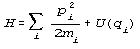Therefore we formulate the

Proposition:

The Hamiltonianis a minimized function of a variational problem which fulfills the equation of motion as well.

Proof:

If we make the coordinate transformations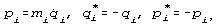, and if we redefine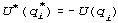we can rewrite the HamiltonianWe see from the second equation that -due to spatial invariance- the Hamiltonian can be looked at as a Lagrangian as well. It follows:Becausewe have a weak minimum for the Hamiltonian .3. The transition from mechanics to thermodynamics

If the assumption is founded that the ergodic hypothesis can be applied to a many particle system then the action integral mean total particle energy in time is identical to the ensemble average or in mathematic language with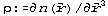the energy formula, W is the probability function and U is the potential. In doing this we loose any time depedent information of the system and we restrict ourselves to determine the total mean amount of the sum of all energy values from the Hamiltonian. On the other hand the description of the system becomes more simple. In principle we are making here the transition from a many particle system ansatz to the conventional thermodynamic description-as we will show in the following (not completely new )

Proposition:
The conventional thermodynamic description can be found by a coordinate transformation from the ensemble average of the Hamiltonian.

Proof: (for one dimensional systems)
From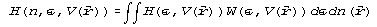follows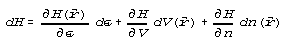with the identifying definitions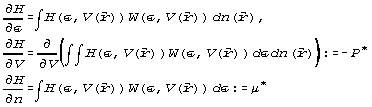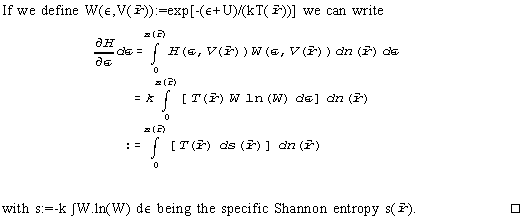It should be said that the total pressureand the total chemical potential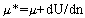are terms which deviate from the conventional barometric measurable pressure P and usual chemical potential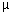because they contain the potential U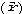additionally. The Shannon entropy derived above approaches the Boltzmann entropy if it goes to a maximum [7,8]. If we extend Gibbs proof  regarding the phase equilibrium in potential fields we see that these total quantities and the temperature are constant over the whole volume of the system in equilibrium, i.e. T=T=constant.This fact allows us to deal with space dependent thermodynamic problems in potential fields generally as hydrostatic or barometric pressure if we identify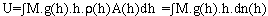for example, comp. , or space dependent phase equilibria of mixtures in fields.

4. The second law as consequence of the least action principle in mechanics

In the last section we showed that the mean Hamiltonian becomes the inner energy in thermodynamics which becomes an extremum as a potential. In this section we will show by an example that the second law follows from the minimum principle of the thermodynamic potentials which itself depends from the least action principle.
We imagine a cycle which includes a irreversible part of the closed path at T=constant and V=constant, see fig.1a . The other part of the cycleis reversible. Because the inner energy U is a potential it holds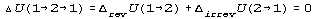Becausedue to minimizing of U during irreversible transitions it follows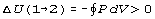and therefrom (because of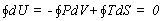)which is the Clausius statement of the second law valid in this case.
It should be said that the analog considerations can be done for a cycle with an irreversible part at P=constant and T=constant by using the enthalpy H as the potential, see fig.1b.

Example:
In order to illustrate the general example given above we present here as a concrete example a simplified version  of a thermodynamic cycle which was proposed originally by Baltzar van Platen, the inventor of the absorbtion refrigerator , see fig.2a-c. This purely isothermal cycle with an periodic irreversibility proceeds in the lower region of the binary P-x-phase diagram of a binary mixture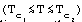. Beginning at state 1 (point 1) at the dew line of the phase diagram, the vapour is compressed reaching state 2 (point 2). In the course of this compression vapour condenses to liquid. At state 2 the volume is split into two parts (see points 2' and 2") by closing a tap, one volume part 2' contains only vapour and the other volume part 2" liquid and vapour.Then both the volumes 2' and 2" are expanded separately up to the initial pressure of the cycle and reach the states 3' and 3" in each compartments.Then the tap between both the compartments is opened, and the initial state1 is reached again on a irreversible path. Fig.2c represents the work area of the van Platen cycle. More of this system and its material conditions in .

According to our understanding of equilibrium thermodynamics the second law is finally a consequence of the principle of least action. Contrary to the conventional point of view it is not an axiom but only a consequence of the mathematics of the problem. The situation is illustrated and summarized in fig. 3 . Because the thermodynamic formalism can describe only the equilibrium, the second law given addionally determines the direction of the process if irreversible states occur.
As shown above this direction depends on the direction of the least action integral of the corresponding many particle system. If the functional has saddle point behavior then the second law in thermodynamics would have to reverse in certain directions of the state space as well in order to maintain consistency. Surprisingly this can happen really if if cyclic varying fields are included into the consideration as we will show in the next web article. We will discuss cases there where the second law reverses.

5.Conclusion
In this article we showed that the thermodynamic potential formalism can be thougth to be stemming from the Hamilton many particle formalism. It could be shown that the Hamiltonian is a potential which net amount minimizes as well as the Lagrangian. In doing the ensemble average of many particles the Hamiltonian becomes the inner energy, whose dynamical behavior is summarized in the extremum principle of the potential which gives the direction of the irreversible process but can not follow numerically the processes in non-equilibrium.  Therefrom, conventional and (as we will see below) non-conventional versions of the second law  follow dependent from the special problem under consideration. Therefore it is easy understandable that many concepts in thermodynamics have an analogon in mechanics. In Tab.1 the most important features are summarized.

Tab.1: analogous features between thermodynamics and mechanics

 mechanics thermodynamics time mean or least action functional ensemble average Hamilton energy inner energy non-minimal state of functional irreversible state Legendre transformations, i.e. L, H Legendre transformations, i.e. U, H, F, G least action principle extremum principle of potentials second variation of functional "second law"

Acknowledgement: Thanks to Remi Cornwall for a constructive critique which helped to fill a hole in my argumentation.

Bibliography:

1) Bronstein-Semendjajew Taschenbuch der Mathematik, Harri Deutsch,Frankfurt, 1984

2) The term total potential stems from van der Waals and Kohnstam
see for example: V.Freise Chemische Thermodynamik BI Taschenbuch1973

3) J.M. Prausnitz, R.N. Lichtenthaler,E.G. de Azevedo
Molecular Thermodynamics of Fluid-Phase Equilibria, second edition,see appendix.1
Prentice Hall, Englewood Cliffs, 1986

4) G. Hilscher Energie im Überfluß, Adolf Sponholtz, Hameln,1981

5) B. van Platen US patent 4.084.484 18.4.1978

6) W.D. Bauer, W. Muschik, Journal of Non-Equilibrium Thermodynamics(in press)
probably vol.23, no.2 or 3, 1998
Second law induced existence conditions for isothermal 2-phase region cyclic processes in binary mixtures

7) K. Huang, Statistical Mechanics, Wiley, New York, 1964
look under 4.1.: Boltzmann´s H-theorem

8) F. Topsoe, Information Theory, Teubner, Stuttgart, 1974

9) R.L. Stratonovich, Nonlinear Nonequilibrium Thermodynamics I, Springer Berlin, 1992fig.1a: isothermal reversible cycle with an irreversible path at V=constant,comp. textfig.1b: isothermal reversible cycle with an irreversible path at P=constant,comp. text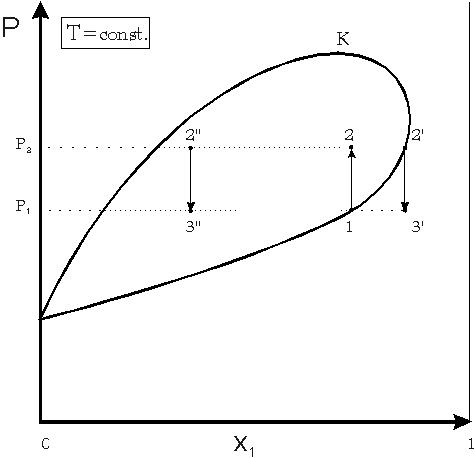fig.2a: simplified van Platen cycle in a P-x-phase diagram of a binary mixture at constant temperature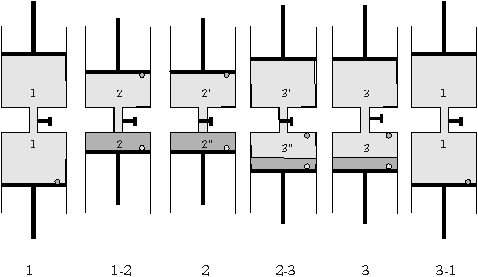fig.2b: simplified van Platen at constant temperature: 1-2 compression,2 splitting the volume, 2-3 separate expansion of both compartement, 3 opening the tap, 3-1 irreversible closing of the cycle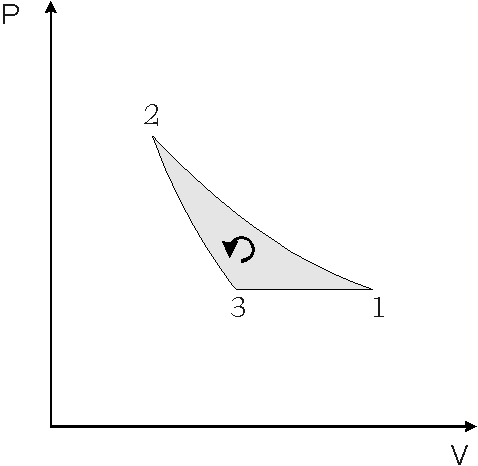fig.2c: total P-V diagram of a simplified van Platen cycle of a binary mixtures of constant temperature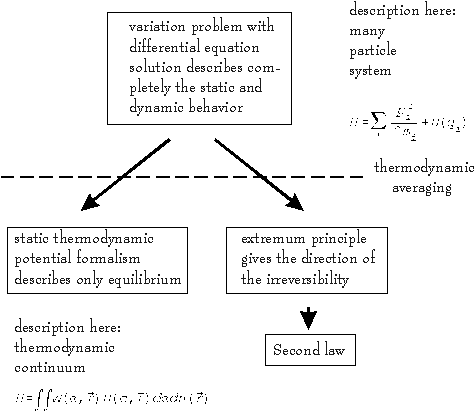fig.3 illustration of derivation of the second law from the principle of least action the direction of the second law is given by the second variation of the least action functional